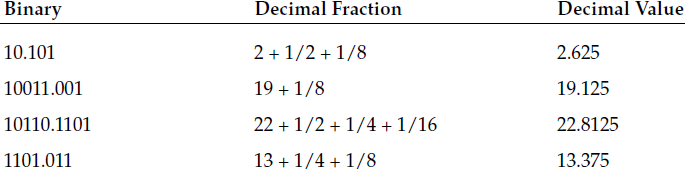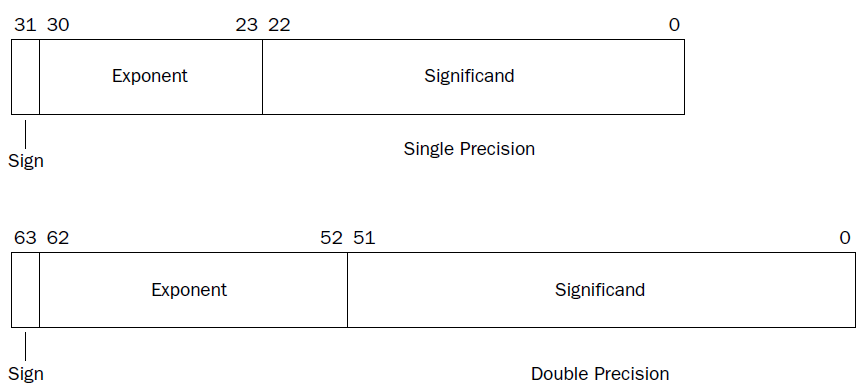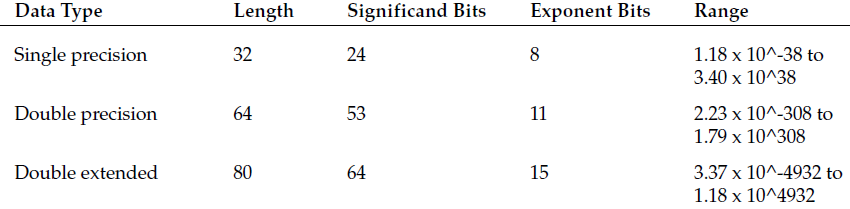﻿ notes7
/*personal notes of renzo diomedi*/

~ 00000111 ~

Floating-Point Numbers

In the decimal world, you are used to seeing values such as 0.159. What this value represents is 0 + 1⁄10 + 5⁄100 + 9⁄1000.
The same principle applies to the binary world.
The coefficient value 1.0101 multiplied by the exponent 2^2 would yield the binary value 101.01, which represents the decimal whole number 5, plus the fraction 0⁄2 + 1/4. This yields the decimal value 5.25.IEEE Standard 754 floating-point formats are used universally to represent real numbers in computer systems.
Intel and AMD have adopted this standard in the IA-32 platform for representing floating-point values.

❑ A sign
❑ A significand
❑ An exponent

The sign bit denotes if the value is negative or positive. A one in the sign bit denotes a negative value, and a zero denotes a positive value.

The exponent is modified to accommodate how many bit positions have been shifted to accomplish this (similar to the scientific notation method). This means that in a normalized value, the significand is always comprised of a one and a binary fraction. The exponent represents the exponent part of the floating-point number. Because the exponent value can be positive or negative, it is offset by a bias value. This ensures that the exponent field can only be a positive unsigned integer. It also limits the minimum and maximum exponent values available for use in the format.

The significand part represents the coefficient (or mantissa) of the floating-point number. The coefficient can be either normalized or denormalized. When a binary value is normalized, it is written with a one before the decimal point.

The IEEE Standard 754 defines two sizes of floating-point numbers:

❑ 32-bits (single-precision)

❑ 64-bits (double-precision)then# fpux
.section .data
value1:
.float 12.34 # directive to create 32-bit singleprecision values
value2:
.double 2353.631 # directive is used to create 64-bit double-precision values
.section .bss
.lcomm data, 8 # label that points to an empty buffer in memory that will be used to transfer a double-precision floating-point value
.section .text
.globl _start
_start:
nop
fstl data # instruction for retrieving the top value on the FPU register stack and placing the value in a memory location
# FSTS for single-precision numbers and FSTL for double-precision numbers
movl \$1, %eax
movl \$0, %ebx
int \$0x80

fpux.s
fpux.o
fpux.exe

C:\>gdb -q users\rnz\desktop\fpux.exe
(gdb) break 1
Breakpoint 1 at 0x401000: file users\rnz\desktop\fpux.s, line 1.
(gdb) run
Starting program: C:\users\rnz\desktop\fpux.exe
Breakpoint 1, start () at users\rnz\desktop\fpux.s:12
12 nop
(gdb) s
13 flds value1
(gdb) x/4b &value1
0x402000 : -92 112 69 65
(gdb) x/4 &value1
0x402000 : 0xa4 0x70 0x45 0x41
(gdb) x/4t &value1
0x402000 : 10100100 01110000 01000101 01000001
(gdb) x/gf &value2
0x402004 : 2353.6309999999999
(gdb) s
14 fldl value2
(gdb) print \$st0
\$1 = 12.340000152587890625 # FIRST ELEMENT IS LOADED IN LOWEST ADDRESS REGISTER
(gdb) print \$st1
\$2 = 0
(gdb) s
15 fstl data
(gdb) print \$st0
\$3 = 2353.63099999999985812 # LAST ELEMENT IS LOADED IN LOWEST ADDRESS REGISTER
(gdb) print \$st1
\$4 = 12.340000152587890625 # PREVIOUS ELEMENT SHIFTS IN NEXT HIGHER ADDRESS REGISTER
(gdb) x/gf &data
0x403000 : 0 # after indtruction FSTL data still set to zero
(gdb) s
16 movl \$1, %eax
(gdb) x/gf &data
0x403000 : 2353.6309999999999 # now after movl \$1 %eax , fpu stack pointer retrieve last element pushed in st0

The FSTL instruction loaded the value in the ST0 register to the memory location pointed to by the data label.